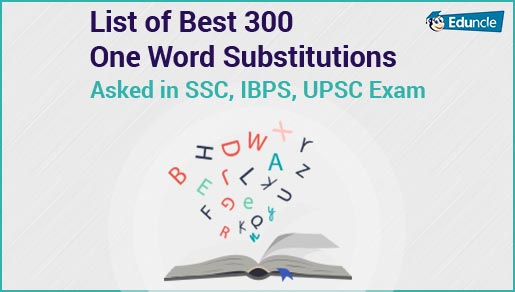Government Jobs FollowRakhi @Eduncle posted an Article
July 6, 2019 • 12:47 pm

# Mixture and Alligation Basic Concepts – Asked in SSC, IBPS, UPSC ExamMixture and Alligation is the most important and common topic in government exams like IBPS, SBI, RRB, SSC exams. It is a part of the quantitative ability section.

The rules of Mixture and Alligation Questions help the candidate to find out the proportion between the two-given commodity.

Mixture and Alligation are two different terms. Before solving mixture and alligations questions you should first know what they actually are. So, let’s start with the definition of Mixture.

Don’t miss it! Benefit yourself by grabbing more useful study stuff to get help in competitive exam preparation. To avail it, please Register Now!

Mixture

The mixture is the mixing of two or more group of different types or two or more items of different types.

When two or more than two substances are in any ratio to produce a product is known as Mixture mean price. The cost price of a unit quantity of the mixture is called the mean price.

Alligations

It deals with how much quantity you want to mix with the two or more items so, the final quantity can be calculated. Alligations are dealing with the quantity.

It is the rule that enables us to find the ratio in which two or more ingredients at the given price must be mixed to produce a mixture of the desired price.

## Mixture & Alligation Rules

Before understanding any new topic, it’s very important that you know the basic concepts of the chapter. So, to clear all your doubts we have mentioned Mixture and Alligation tricks and formulas with their examples to solve the question in a competitive exam in minimal time. Lets start with the mixture and allegation examples.

### Rule:1

Let’s suppose if the cost price of the cheaper item is ‘Rs. C’ and the cost price of the dearer item be ‘Rs. D’ and the average or the mean price of a mixture be ‘m’ then,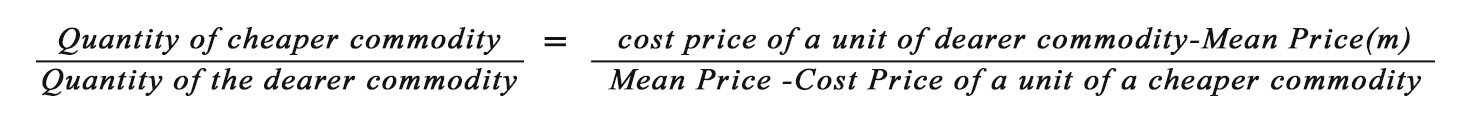You can also represent the equation as:Hence,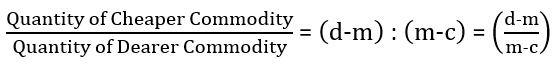Have a look on to 300+ Useful Idioms & Phrases to Crack SSC & IBPS English Exams!

Question: In what proportion must tea at Rs. 10 per kilogram be mixed with tea at Rs. 14 per kilogram. So, that the mixture be worth Rs. 12 per kilogram?

Solution: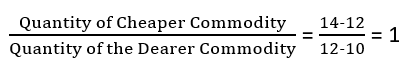### Suppose, there are two bowls which are filled with water and milk. In container A the ratio of water and milk is a:b and in container B the ratio of water and milk is c:d. If both the containers are emptied into a third container then, the ratio of milk to water in the third container will be as follow: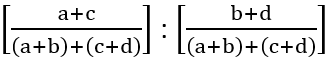Question: There are two containers which are filled with water and milk. The first container is filled in the ratio of 3:1 and the second container is filled with 5:2. If, both the containers mixture is mixed-up then, the ratio of milk to the water in the mixture will be?

Solution:

Portion of milk in first container = 3/(3+1)=3/4

Portion of water in first container = 1/(3+1)=1/4

Same way,

Portion of milk in second container = 5/(5+2)=5/7

Portion of water in second container = 2/(5+2)=2/7

Therefore, the ratio of water and the milk in the third container will be = 3/4 + 5/7 : 1/4 + 2/7 = 41/28 : 15/28

Get a taste of SUCCESS by cracking the competitive exams like IBPS, SSC, RRB, UPSC, etc. SUBSCRIBE US and discover the exciting strategies!

### Rule:3

Repeated Dilutions

Suppose a container contains ‘x’ unit of a liquid from which ‘y’ units are taken out and replaced by water. After an operation, the quantity of pure liquid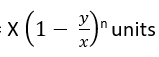Question: A container contains 40 litres of milk. From this container, 4 litres of milk was taken out and replaced by water. This process was repeated further two times. How much milk is now contained by the container?

Solution: Amount of milk left after 3 operations will be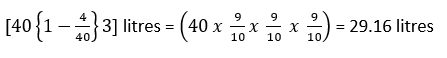This brings us to the end of our article. Hope you are now aware of the mixture and alligation tricks, formulae and concepts to solve the questions easily.

If you have any queries or doubts regarding the above-mentioned information, then you can ask us by leaving a comment in the section belw. We'll try to get in touch with you as soon as possible. Thanks!

Happy Preparing Aspirants!

You Might Also Like